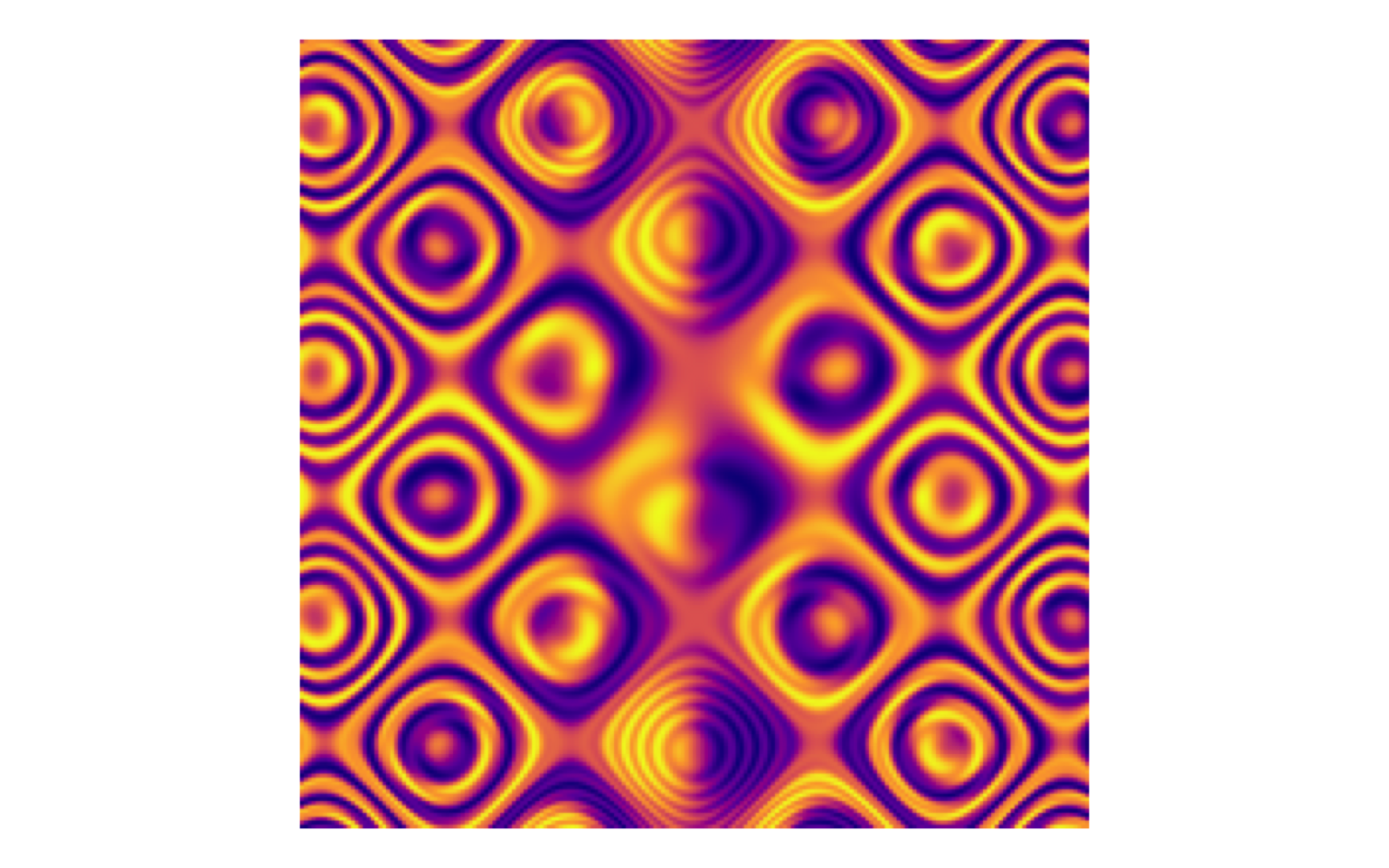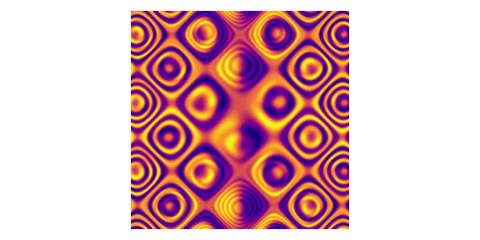# Making an animated contour plotEarlier this week Mike Bostock tweeted a interesting looking contour plot with a link to edit the formula and manipulate the graphic using D3.js.

I decided I would attempt to recreate the image using ggplot2, and animate it using the new gganimate package.

## Creating the data

I started by creating a data frame with all the combinations of x and y on a grid between -10 and 10, in intervals of 0.1. Then I defined a third variable, z as a function of x and y, using the same equation as originally used here.

plot_data <- crossing(x = seq(-10, 10, 0.1), y = seq(-10, 10, 0.1)) %>%
mutate(z = sin(sin(x * (sin(y) - cos(x)))) - cos(cos(y * (cos(x) - sin(y)))))

plot_data
#> # A tibble: 40,401 x 3
#>        x     y       z
#>    <dbl> <dbl>   <dbl>
#>  1   -10 -10   -1.77
#>  2   -10  -9.9 -0.950
#>  3   -10  -9.8 -0.275
#>  4   -10  -9.7 -0.138
#>  5   -10  -9.6  0.0279
#>  6   -10  -9.5 -1.01
#>  7   -10  -9.4 -1.80
#>  8   -10  -9.3 -1.28
#>  9   -10  -9.2 -0.564
#> 10   -10  -9.1 -0.227
#> # … with 40,391 more rows

Now we can use that data to make the image!

## Create the plot

To create the plot, we are basically making a heat map of sorts, where the fill is defined by the newly calculated z variable. I use geom_raster because it offers speed improvements over geom_tile when all tiles are the szme size, which they are in this case. Given that we have a lot of tiles, I’ll take the speed!

ggplot(plot_data, aes(x = x, y = y)) +
geom_raster(aes(fill = z), interpolate = TRUE, show.legend = FALSE) +
scale_fill_viridis_c(option = "C") +
coord_equal() +
theme_void()Pretty good! But then, Jeff Baumes upped the stakes!

Well now I can’t not animate it. So…

To create an animated plot, we need a time variable, t. We start by creating a data frame similar to the one we created above. It includes all combinations of x and y from -10 to 10 in increments of 0.1, but now those values are also cross with the time variable, t, which goes from 0 to 5 in increments of 0.1. The z variable, which will still be our fill color, is now a function of x, y, and t, as in Jeff’s example. Finally, we can use the same ggplot2 code as above, with the addition of the transition_time function from gganimate.

t_lookup = data_frame(t = c(seq(0, 5, 0.1), seq(4.9, 0, -0.1)),
t2 = seq(0, 10, 0.1))

plot_data <- crossing(x = seq(-10, 10, 0.1), y = seq(-10, 10, 0.1),
t2 = seq(0, 10, 0.1)) %>%
left_join(t_lookup, by = "t2") %>%
mutate(z = sin(sin(x * (sin(y + t) - cos(x - t)))) - cos(cos(y * (cos(x - t) - sin(y + t)))))

ggplot(plot_data, aes(x = x, y = y)) +
geom_raster(aes(fill = z), interpolate = TRUE, show.legend = FALSE) +
scale_fill_viridis_c(option = "C") +
coord_equal() +
theme_void() +
transition_time(t2)And there we have it! With just one extra variable and one additional line of code for our plot, we have an animated contour plot!###### Senior Psychometrician

I’m a psychometrician and educational assessment researcher who loves using R to learn from data.